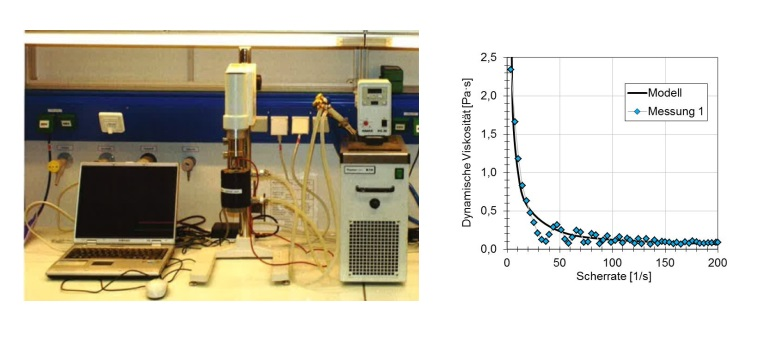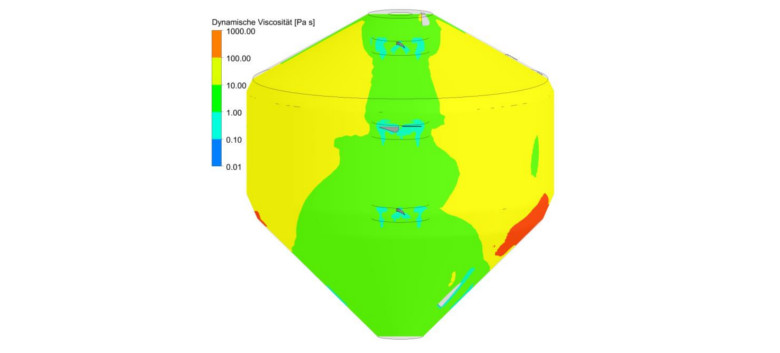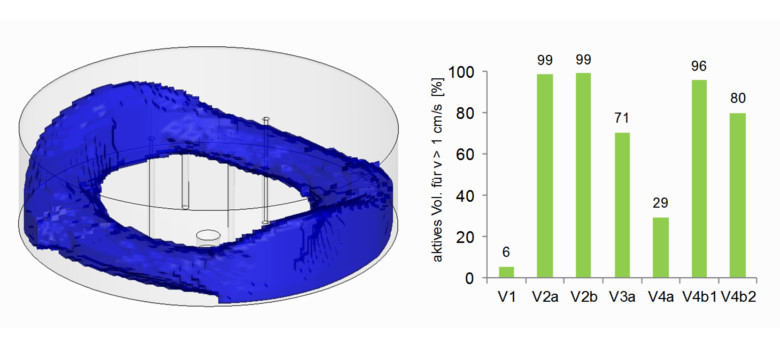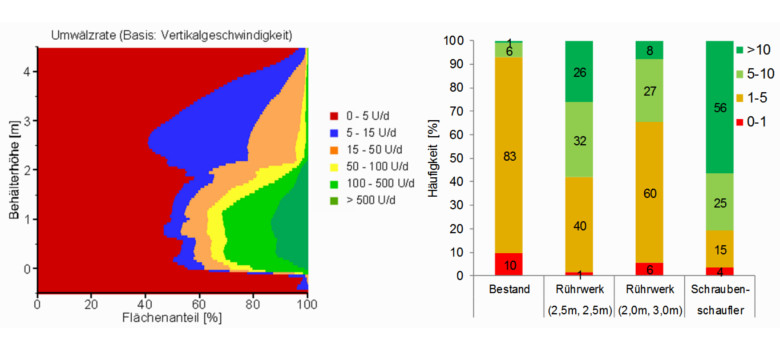Simulation of Anaerobic Digesters

What are the benefits of hydrograv simulations?

• Maximization of the active volume
• Maximization of the circulation rate
• Deterministic comparision of variants and optimization of various mixing systems like pumps, screw pumps, agitators or gas injection

Example 1: Measurement and simulation of the actual sludge viscosity to increase the forecasting reliabilty

• Selection and parameterization of a appropiate rheology model based on laboratory measurements using a viscometerFigure: Laboratory with measuring technology to determine viscosity (left) and measured viscosity as a function of shear rate and adapted rheology model (right).Figure:   Simulated dynamic viscosity in a digester with three-bladed agitator.

Example 2: Visualisation of processes

• Streamlines visualisise the flow path and show areas with hydraulic deficits, e.g. short circuitsFigure: Streamlines with velocities.

#### Example 3: Determination of areas with acitve volume

• quantitative determination of areas with velocities greater than 1 cm/sFigure: Visualization and analysis of the active volume in a digester for different geometrical and operational variants.

Example 4: Detailed deterministic analysis of the actual circulation rate

• Circulation rate as a function of the flow velocity, e.g. as a funcion of the tank height or as volume fractionFigure: Analysis of the circulation rate along the tank height (left) and als fraction of the total volume (right).